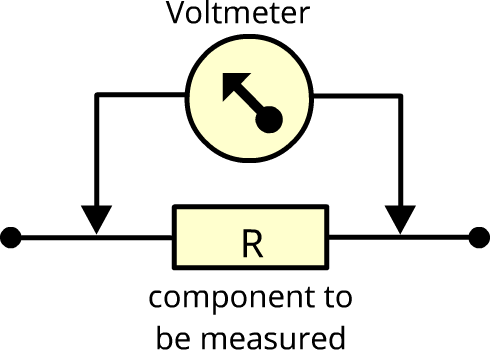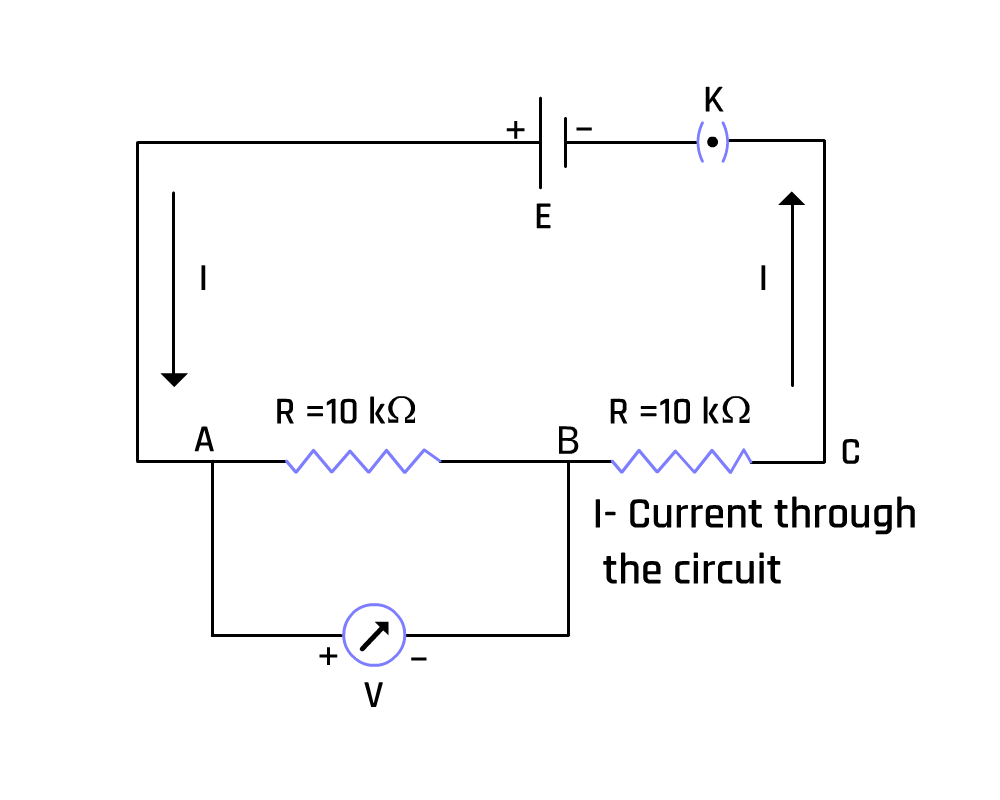Voltage Measuring Device has Non Infinite Resistance

# Objective

An ideal voltage-measuring device has infinite resistance. But in practical cases, it is not possible. Here, our aim is to demonstrate a non-ideal voltage measuring device has non-infinite resistance.

# Theory

A voltmeter is a device to measure the potential difference between two points. It measures the potential difference between two points by connecting voltmeter terminals to those two points without breaking the wire.Figure 1: Measurement using voltmeter
A voltmeter is connected parallel to the location being measured. The current passing through the voltmeter must be zero for the exact measurement of voltage.  So, the resistance of an ideal voltmeter is infinite.Iv = current through the voltmeter

(V = voltmeter reading, R = resistance of voltmeter)
When R is infinite, Iv becomes zero.
In practical case, there is no ideal voltmeter. Each voltmeter has non-infinite resistance.Figure 2: Circuit diagram

• Consider the circuit.

I = current through the circuit.

Rv = resistance of voltmeter.

E = voltage of the cell.

R = resistance of 10 kilo ohm.

Voltmeter measures potential difference across AB.Current through the circuitThe voltage across AB is measured using a voltmeter.This equation can calculate the resistance of the voltmeter.# Learning Outcomes

• Students understand concepts and the differences between ideal and non-ideal voltmeters.